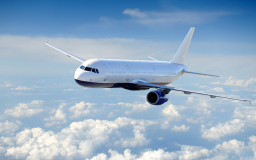# Difference 69354

An airplane flies at a speed of 920 km/h at an altitude of 11 km above the surface of the Earth, where the air density is p = 0.36 kg/m3. Determine the pressure difference above and below the plane's wing if the top of the plane is 10% longer than the bottom.

Δ =  2468.67 Pa

### Step-by-step explanation:Did you find an error or inaccuracy? Feel free to write us. Thank you!

Tips for related online calculators
Need help calculating sum, simplifying, or multiplying fractions? Try our fraction calculator.